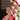# 图论入门

January 02, 2018

``````第一行包含三个整数N、M、S，分别表示点的个数、有向边的个数、出发点的编号。

（文字来源：Luogu

## 二维数组？

``````#include <bits/stdc++.h>
using namespace std;

const int MAXN = 1e4+10;

int mapn[MAXN][MAXN];

int N,M,S;

{
mapn[a][b]=weight;
}

int main()
{
cin>>N>>M>>S;
for(int i=1; i<=M; i++)
{
int u,v,w;
cin>>u>>v>>w;
}
}``````

## vector 存边法

``````#include <bits/stdc++.h>

using namespace std;

const int MAXN = 1e4+10;

struct Edge
{
int from;
int to;
int weight;
Edge(int f,int t,int w)
{
this->from=f;
this->to=t;
this->weight=w;
}
Edge(){}
};

vector<Edge> mapn[MAXN];
int N,M,S;
// 加边
{
mapn[a].push_back(Edge(a,b,w));
}

// main()与上边一样，省略``````

### 有什么优点？

• 便于遍历。想从某一个点出发遍历他所连着的点只需要把这个点的vector遍历一遍即可。
• 省内存。可以计算出这个程序存储图所用空间最大大致为`边数*12/(1024*1024)` 即6M左右

## 链式前向星

``````const int MAXM = 5e5 + 10;
const int MAXN = 1e4 + 10;
struct Edge
{
int to;
int next;
}edges[MAXM];

int first[MAXN];

int edges_stamp=0;

// 需要在main()中 memset(first,-1,sizeof(first));